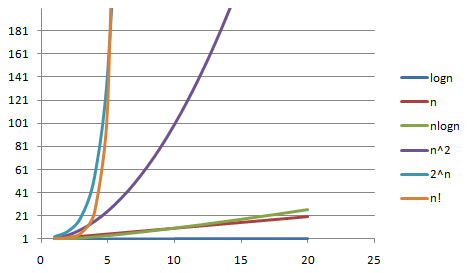# 算法（一）时间复杂度

### 3.大O表示法

1.用常数1来取代运行时间中所有加法常数。
2.修改后的运行次数函数中，只保留最高阶项
3.如果最高阶项存在且不是1，则去除与这个项相乘的常数。

  int sum = 0,n = 100; //执行一次
sum = (1+n)*n/2; //执行一次
System.out.println (sum); //执行一次 

for(int i=0;i<n;i++){
//时间复杂度为O(1)的算法
...
}

int number=1;
while(number<n){
number=number*2;
//时间复杂度为O(1)的算法
...
}

  for(int i=0;i<n;i++){
for(int j=0;j<n;i++){
//复杂度为O(1)的算法
...
}
}

  for(int i=0;i<n;i++){
for(int j=i;j<n;i++){
//复杂度为O(1)的算法
...
}
}

n+(n-1)+(n-2)+(n-3)+……+1
=(n+1)+[(n-1)+2]+[(n-2)+3]+[(n-3)+4]+……
=(n+1)+(n+1)+(n+1)+(n+1)+……
=(n+1)n/2
=n(n+1)/2
=n²/2+n/2

f(n)=nlogn时，时间复杂度为O(nlogn)，可以称为nlogn阶。
f(n)=n³时，时间复杂度为O(n³)，可以称为立方阶。
f(n)=2ⁿ时，时间复杂度为O(2ⁿ)，可以称为指数阶。
f(n)=n!时，时间复杂度为O(n!)，可以称为阶乘阶。
f(n)=(√n时，时间复杂度为O(√n)，可以称为平方根阶。

### 4.复杂度的比较

nlogn√nnlogn2ⁿn!
522102532120
10333010010243628800
50572502500约10^15约3.0*10^64
10061060010000约10^30约9.3*10^157
100093190001000 000约10^300约4.0*10^2567O(1)<O(logn)<O(n)<O(nlogn)<O(n²)<O(n³)<O(2ⁿ)<O(n!)


《大话数据结构》
《挑战程序设计竞赛2》
《算法》08-26758909-2025万+
11-232万+
07-18663
06-24268
09-057万+
10-282万+
05-233万+
12-029040
06-261494
09-2326万+
08-15967
10-282万+
04-02879
06-23660
01-0140
01-2152
11-027088